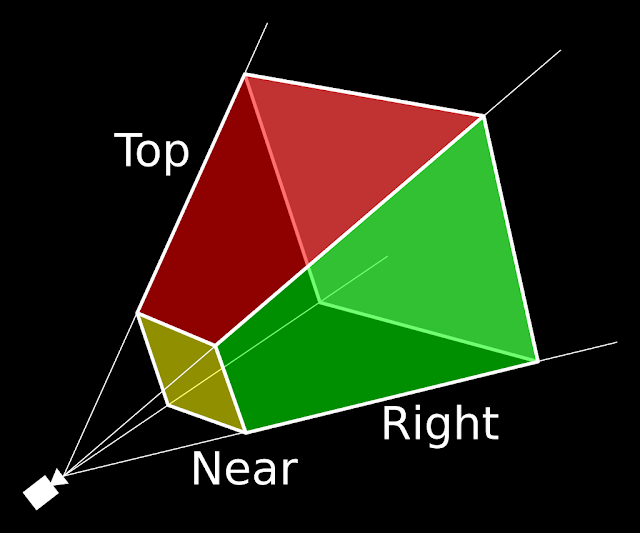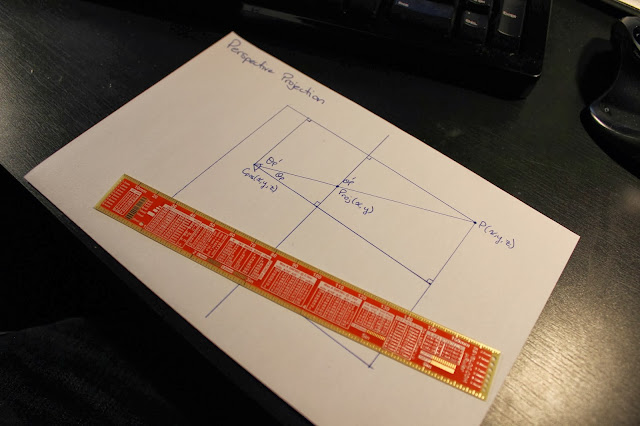## Wednesday, January 1, 2014

### 3D Perspective Projection

I have wanted to write a 3D rendering engine for years and I have finally decided to spend some time on the problem. I decided to implement a simplistic rendering engine that supports the following basic features:
• 3D Perspective Projection (far objects are smaller than near objects)
• Triangular Polygons for Rendering
• Simplistic Lighting Model
• Colored Polygon Faces (no texturing)
The largest hurdle for me was getting past perspective projection. I did some research about matrix transformations and got caught up quite deeply in mathematical abstraction. It was the image below that really set my mind in motion. I decided to take a step back and use some simple high school trigonometry to solve the problem.Leon Battista Alberti and Perspective Projection
I have spent approximately 13 hours on this project and the results so far are fantastic looking. This entire experiment has given me great insights into the way 3D geometry is rendered onto a 2D plane.The Classic Utah Teapot :]
In this article I will show you the various steps that I have taken to render this image. I will focus on the mathematics behind perspective projection that I used to arrive at the teapot rendering above. The image is not perfect, but I am happy with the results.

### Perspective Projection

The largest stumbling block for me was rendering the geometry onto the plane that is my display. As I mentioned earlier, most explanations of perspective projection start out with camera matrix transforms. I found this very abstract. For the scope of this project I wanted to ignore the concepts of world coordinates, camera coordinates and so on. I started out with the assumption that the geometry that I wish to render is already located in front of the camera.

The viewing frustum is the most basic concept of perspective projection. It defines the plane onto which your 3D geometry will be projected (labelled "near" in the image below).Viewing Frustum
The viewing frustum is defined by the users field of view. Field of view is specified in degrees and then in combination with the width of the viewport in pixels we can determine the users distance from
the screen.Field of View
The field of view is a user defined parameter of the rendering engine. I set will set mine to 100 degrees. Let's say that the width of the viewport for this example is 800 pixels. We can calculate the users distance from the plane as follows:
$$\tan(\theta _{\mathrm{fov}} /2) = \mathrm{\frac{w/2}{d}} \\ \mathrm{d} = \mathrm{\frac{w/2}{\tan(\theta _{\mathrm{fov}} / 2)}} \\ \mathrm{d} = \mathrm{\frac{800/2}{\tan(100 / 2)}} \\ \mathrm{d} = 335.639$$
Next I decided to work on projecting the actual points onto the plane. I started out on a piece of paper with a neat little drawing and started to realize that it is quite an easy feat.Projection Sketch
This is an extremely simple problem to solve. We know the camera position and we know the point that we wish to plot. Now it is just a matter of solving for the angle of projection and then using the concept of similar triangles to resolve the point on the screen. The camera position will be (0, 0, d) where d is the distance that you calculated earlier.Perspective Projection Diagram
I will demonstrate how to solve the y-component of the projected point first. The same formula will then be applied to the x-component. First we must solve for the angle of projection in the y-axis.
$$\tan(\theta_{\mathrm{proj}}) = \mathrm{\frac{P_{y}}{P_{z} + C_{z}}} \\ \theta_{\mathrm{proj}} = \tan^{-1}\left(\frac{P_{y}}{P_{z} + C_{z}}\right)$$
We will leave it in this form and solve for the y component of the projected point.
$$\tan(\theta_{\mathrm{proj}}) = \mathrm{\frac{Proj_{y}}{C_{z}}}$$
We can now substitute the equation for the projected angle into the equation for the y-component of the projected point.
$$\tan\left(\tan^{-1}\left(\frac{P_{y}}{P_{z} + C_{z}}\right)\right) = \mathrm{\frac{Proj_{y}}{C_{z}}} \\ \frac{P_{y}}{P_{z} + C_{z}} = \mathrm{\frac{Proj_{y}}{C_{z}}} \\ \mathrm{Proj_{y}} = \frac{C_{z}P_{y}}{P_{z} + C_{z}}$$
That's it! The formula is quite simple and the same can be applied to the x-component of the projected point as well.
$$\mathrm{Proj_{x}} = \frac{C_{z}P_{x}}{P_{z} + C_{z}}$$
At this point, you will be able to plot points onto your screen!An early test render :]
I have implemented the rendering engine in C# using Mono on Linux. I am using the built-in System.Drawing namepsace that is based on Cairo to render the polygons. I will be publishing another article that explains how I modelled the geometry and the concepts behind lighting.